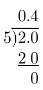Refer to our Texas Go Math Grade 6 Answer Key Pdf to score good marks in the exams. Test yourself by practicing the problems from Texas Go Math Grade 6 Module 2 Quiz Answer Key.

2.1 Classifying Rational Numbers

Question 1.
Five friends divide three bags of apples equally between them.
Write the division represented in this situation as a fraction.
As 5 friends divide 3 bags of apples equally between them, we can write it as fraction as:
$$\frac{3}{5}$$

Write each rational number as $$\frac{a}{b}$$

Question 2.
5$$\frac{1}{6}$$ __________________
Firstly, we will write the whole number as a sum of ones
Secondly, we will use the denominator to find corresponding fractions for ones.
Thirdly, we will calculate sum of numerators!
To reach our final solution: improper fraction.
5$$\frac{1}{6}$$ = 1 + 1 + 1 + 1 + 1 + $$\frac{1}{6}$$
= $$\frac{6}{6}+\frac{6}{6}+\frac{6}{6}+\frac{6}{6}+\frac{6}{6}+\frac{1}{6}$$
= $$\frac{31}{6}$$

Question 3.
– 12 __________________
Number – 12 can be written as fraction as:
– 12 = – $$\frac{12}{1}$$

Determine if each number is a whole number, integer, or rational number. Include all sets to which each number belongs.

Question 4.
– 12 __________________
Number – 12 belongs to set of Integers
Therefore, it also belongs to set of Rational numbers

Question 5.
$$\frac{7}{8}$$ __________________
Number $$\frac{7}{8}$$ belongs to set of Rational Numbers

2.2 Identifying Opposites and Absolute Value of Rational Numbers

Question 6.
Graph – 3, 1$$\frac{3}{4}$$, – 0.5, and 3 on the number line.Firstly, we transform fractions into decimals!
1$$\frac{3}{4}$$ = 1 + $$\frac{3}{4}$$ = 1 + 0.75 = 1.75Division: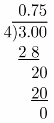Question 7.
Find the opposite of $$\frac{1}{3}$$ and – $$\frac{7}{12}$$ __________
As i mentioned before, opposite of any number is number with added – sign on left side!
Opposite of $$\frac{1}{3}$$ is –$$\frac{1}{3}$$
Opposite of – $$\frac{7}{12}$$ is $$\frac{7}{12}$$
– $$\frac{1}{3}$$ and $$\frac{7}{12}$$

Question 8.
Find the absolute value of 9.8 and –$$\frac{7}{8}$$ __________
Absolute value of some number is its distance from 0 on the number line!
|9.8| = 9.8
|- $$\frac{10}{3}$$| = $$\frac{10}{3}$$
9.8 and $$\frac{10}{3}$$

2.3 Comparing and Ordering Rational Numbers

Question 9.
Over the last week, the daily low temperatures in degrees Fahrenheit have been – 4, 6.2, 18$$\frac{1}{2}$$, – 5.9, 21, – $$\frac{1}{4}$$, and 1.75. List these numbers in order from greatest to least.
First thing will be writing fractions as equivalent decimals!
18$$\frac{1}{2}$$ = 18 + $$\frac{1}{2}$$ = 18 + 0.5 = 18.5
– $$\frac{1}{4}$$ = – 0.25

Now we know that number 21 is greater than 18.5 which is greater than 6.2 which is greater than 1.75 which is
greater than – 0.25 which ¡s greater than – 4 which is greater than – 5.9.
One last thing to do is to return decimals to given expressions and write it in order from greatest to least!
21, 18$$\frac{1}{2}$$, 6.2, 1.75, –$$\frac{1}{2}$$, – 4, – 5.9

Division:
$$\frac{1}{2}$$ is very easy, so we won’t show it!Essential Question

Question 10.
How can you solve problems by ordering rational numbers from least to greatest?
Firstly, we have to convert all expressions into same, as shown in examples above, we can work with fractions or
decimals, depending on given terms!
Secondly, we compare our decimals/fractions and order them from least to greatest!
Note that we compare numerators when dealing with fractions. That works because we get them to have same denominator!
One last thing to do is to return them in given format and write a final solution!

Texas Go Math Grade 6 Module 2 Mixed Review Texas Test Prep Answer Key

Selected Response

Question 1.
Suki split five dog treats equally among her six dogs. Which fraction represents this division?
(A) $$\frac{6}{5}$$ of a treat
(B) $$\frac{5}{6}$$ of a treat
(C) $$\frac{1}{5}$$ of a treat
(D) $$\frac{1}{6}$$ of a treat
As Suki splits 5 dog treats among 6 dogs.
Each dog will get
$$\frac{5}{6}$$ treats

(B) $$\frac{5}{6}$$ of a treat

Question 2.
Which set or sets does the number 15 belong to?
(A) whole numbers only
(B) rational numbers only
(C) integers and rational numbers only
(D) whole numbers, integers, and rational
The number 15 belongs to set of Whole numbers and therefore to set of Integers and Rational numbers.
We can say that because we know that set of Whole numbers is included in set of Integers, and set of Integers is included in set of Rational numbers.

Question 3.
Which of the following statements about rational numbers is correct?
(A) All rational numbers are also whole numbers.
(B) All rational numbers are also integers.
(C) All rational numbers can be written in the form $$\frac{a}{b}$$.
(D) Rational numbers cannot be negative.
Statement that all rational numbers are also whole numbers is surely incorrect!
For example, 0.5 is a decimal, which is not whole number!

Statement that all rational numbers are also integers is also incorrect!
We can use same example from last statement to prove it.

Statement that all rational numbers can be written in form of $$\frac{a}{b}$$ is correct!
Really, it is…take a look at some examples that prove our theory:
0.5 = $$\frac{1}{2}$$
– 5 = $$\frac{-5}{1}$$
5 = $$\frac{5}{1}$$

Statement that rational numbers cannot be negative is incorrect!
We can simply take a look on Venn’s diagram(which we worked in previous lecture) to convince ourselves that this is false statement!

(C) All rational numbers can be written in the form $$\frac{a}{b}$$.

Question 4.
Which of the following shows the numbers in order from least to greatest?
(A) –$$\frac{1}{5}$$, – $$\frac{2}{3}$$, 2, 0.4
(B) 2, –$$\frac{2}{3}$$, 0.4, – $$\frac{1}{5}$$
(C) – $$\frac{2}{3}$$, 0.4, – $$\frac{1}{5}$$, 2
(D) – $$\frac{2}{3}$$, – $$\frac{1}{5}$$, 0.4, 2
Let’s transform all the given numbers into fractions first and make them have 15 as common denominator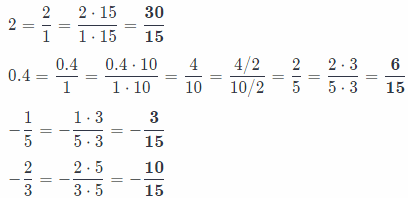Now, we compare their numerators to find out their order
30, 6, – 3, – 10
Number – 10 is less than – 3 which is less than 6 which is Less than 30.
So, now we know order of numerators, and therefore we know order of whole fractions

So, numbers in order from least to greatest are:
$$-\frac{10}{15},-\frac{3}{15}, \frac{6}{15}, \frac{30}{15}$$
Now, we can return them in given form and get:
– $$\frac{2}{3}$$, – $$\frac{1}{5}$$, 0.4, 2

(D) – $$\frac{2}{3}$$, – $$\frac{1}{5}$$, 0.4, 2

Question 5.
What is the absolute value of – 12.5?
(A) 12.5
(B) 1
(C) – 1
(D) – 12.5
Absolute value of some number is its distance from 0 on the number line!
|- 12.5| = 12.5

(D) – 12.5

Question 6.
Which number line shows –$$\frac{1}{4}$$ and its opposite?
(A)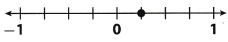(B)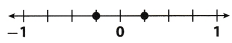(C)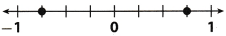(D)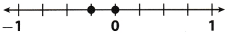Let’s draw our number line to find out the solution: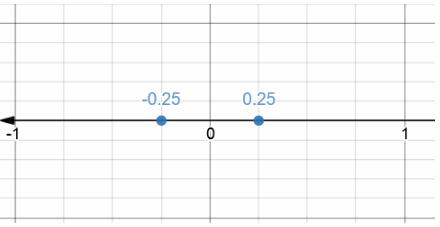Note that we used 0.25 instead of $$\frac{1}{4}$$ since these expressions are equivalent!

(B)Question 7.
Horatio climbed to the top of a ladder that is 10 feet high. What is the opposite of Horatio’s height on the ladder?
(A) – 10 feet
(B) 10 feet
(C) 0 feet
(D) $$\frac{1}{10}$$ foot
Opposite of some number is that same number with added – sign on a left side.
So, in our case, opposite of 10 is -10 feet.

(A) – 10 feet

Gridded Response

Question 8.
The heights of four students in Mrs. Patel’s class are 5$$\frac{1}{2}$$feet, 5.35 feet, 5$$\frac{4}{10}$$ feet, and 5.5 feet. What is the height in feet of the shortest student written as a decimal?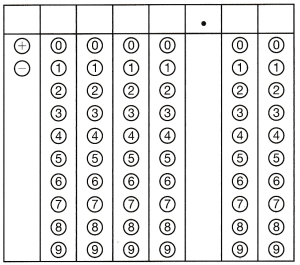5$$\frac{1}{2}$$ = 5 + $$\frac{1}{2}$$ = 5 + 0.5 = 5.5
5$$\frac{4}{10}$$ = 5 + $$\frac{4}{10}$$ = 5 + $$\frac{4 / 2}{10 / 2}$$ = 5 + $$\frac{2}{5}$$ = 5 + 0.4 = 5.4